Students must start practicing the questions from CBSE Sample Papers for Class 10 Maths with Solutions Set 4 are designed as per the revised syllabus.

## CBSE Sample Papers for Class 10 Maths Standard Set 4 with Solutions

Time Allowed: 3 Hours
Maximum Marks: 80

General Instructions:

1. This Question Paper has 5 Sections A-E.
2. Section A has 20 MCQs carrying 1 mark each
3. Section B has 5 questions carrying 02 marks each.
4. Section C has 6 questions carrying 03 marks each.
5. Section D has 4 questions carrying 05 marks each.
6. Section E has 3 case based integrated units of assessment (04 marks each) with sub-parts of the values of 1, 1 and 2 marks each respectively.
7. All Questions are compulsory. However, an internal choice in 2 Qs of 5 marks, 2 Qs of 3 marks and 2 Questions of 2 marks has been provided. An internal choice has been provided in the 2 marks questions of Section E
8. Draw neat figures wherever required. Take π =22/7 wherever required if not stated.

Section – A
(Section A consists of 20 questions of 1 mark each.)

Question 1.
If x = 22 × 33 × 72, y = 23 × 32 × 5 × 7, then HCF (x, y) is: (1)
(a) 250
(b) 252
(c) 160
(d) 140
(b) 252

Explanation:
HCF(x, y) = 22 × 32 × 72 i.e. 252

Question 2.
If the lines represented by 3x + 2py = 2 and 2x + 5y + 1 = 0 are parallel, then the value of p is: (1)
(a) $$\frac{15}{4}$$
(b) 13
(c) 12
(d) $$\frac{19}{2}$$
(a) $$\frac{15}{4}$$

Explanation:
Since the given lines are parallel,
So, $$\frac{3}{2}$$ = $$\frac{2p}{5}$$ ≠ $$\frac{2}{1}$$
⇒ p = $$\frac{15}{4}$$

Question 3.
The value of x and y, x + y = 3 and 7x + 6y = 2 are: (1)
(a) x = 16, y = 9
(b) x = 8, y = 5
(c) x = 2, y = 15
(d) x = -16, y = 19
(d) x = – 16, y = 19

Explanation:
x + y = 3 gives, y = 3 – x …(i)
So, 7x + 6y = 2 gives 7x + 6(3 – x) = 2
⇒ 7x + 18 – 6x = 2
i.e. x = – 16
From (i), y = 3 + 16 = 19
Thus, x = – 16 and y = 19 is the required solution.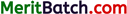Question 4.
Form a quadratic polynomial, whose zeros are -3 and 5. (1)
(a) x2 – 2x – 15
(b) 3x2 + 5
(c) x2 – 3x – 14
(d) x2 + 2x + 15 1
(a) x2 – 2x – 15

Explanation:
Since, α = – 3 and β = 5
Then, α + β = -3 + 5 = 2
α β = -3 × 5 = -15
Hence, x2 – (α + β) x + α β = 0
x2 – 2x – 15 =0,

Question 5.
The distance between the points (0, 5) and (-5, 0) is: (1)
(a) 5$$\sqrt{2}$$
(b) 3$$\sqrt{2}$$
(c) $$\sqrt{3}$$
(d) 7
(a) 5$$\sqrt{2}$$

Explanation:
Distance =$$\sqrt{(- 5 – 0)^2 + (0 – 5)^2}$$
= $$\sqrt{25+25}$$ = $$\sqrt{50}$$ = $$5 \sqrt{2}$$

Question 6.
For what values of ‘a’, does the quadratic equation x2 – ax + 1 = 0 not have real roots? (1)
(a) (2,1)
(b) (0, 1)
(c) (- 2, 2)
(d) (- 1,1)
(c) (- 2, 2)

Explanation:
Given, equation is x2 – ax + 1 = 0
On comparing the equation with Ax2 + Bx + C = 0, we get
A = 1, B = – a and C = 1
Then, D = B2 – 4AC
= (- a)2 – 4 × 1 × 1
= a2 – 4
For no real roots
D < 0
Then, a2 – 4 < 0
a2 < 4
– 2 < a < 2Question 7.
The perimeter of the triangle AOB with vertices A(4, 0), 0(0,0) and B(0, 3) is: (1)
(a) 10 units
(b) 5 units
(c) 12 units
(d) 4 units
(c) 12 units

Explanation:
Given, coordinates of ∆AOB as A(4, 0), O(0, 0) and B(0, 3)
Perimeter of ∆AOB
= Length of AB + length of OA + length of OB
= $$\sqrt{(4-0)^2+(0-3)^2}+\sqrt{(4-0)^2+(0-0)^2}+\sqrt{(0-0)^2+(3-0)^2}$$
= $$\sqrt{16+9}+\sqrt{4^2}+\sqrt{3^2}$$
= 5 + 4 + 3 = 12 units

Question 8.
If p and q are the roots of the quadratic equation x2 + px – q = 0, then find the values of p and q. (1)
(a) p = 4, q = 5
(b) p = 3, q = -2
(c) p = 1, q = 1
(d) p = – 1, q = 2
(d) p = – 1, q = 2

Explanation:
Given, quadratic equation is x2 + px – q =0
Here, sum of roots i.e.
p + q = $$\frac{-p}{1}$$
⇒ 2 p = -q
and product of roots,
pq = $$\frac{-q}{1}$$
⇒ P =- 1
Then, q = 2
Hence, the values of p and q are – 1 and 2 respectively.

Question 9.
In the figure, if ∠A = ∠B and AD = BE. Then DE is Parallel to: (1)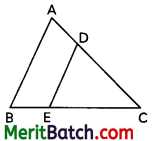(a) BE
(b) EC
(c) AC
(d) AB
(d)AB

Explanation:
In A∆ABC, ∠A = ∠B gives, BC = CA
As AD = BE so, DC = CE
⇒ $$\frac{CD}{AD}$$ = $$\frac{CE}{BE}$$
Hence, by the converse of Thales’ theorem DE || AB.

Question 10.
The distance between two parallel tangents to a circle of radius 5 cm is: (1)
(a) 5 cm
(b) 8 cm
(c) 10 cm
(d) 9 cm
(c) 10 cm

Explanation:
Here, AB and CD are 2 parallel tangents to a circle with centre O.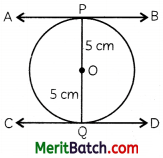Then, distance between two parallel tangents
= OP+ OQ
= 5 + 5 = 10 cmQuestion 11.
The point (5, -3) lies in : (1)

Explanation:
First quadrant, X = positive and Y = positive
Second quadrant, X = negative and Y = positive
Third quadrant, X = negative and Y = negative
Fourth quadrant, X = positive and Y = negative
(5,-3) has X = 5, positive and Y = 3, negative
∴ The point lies in the fourth quadrant.

Question 12.
In the figure, ∠APB = 90°. The length of OP is: (1)(a) 2r
(b) $$\sqrt{3}$$r
(c) 4r
(d) $$\sqrt{2}$$r
(d) $$\sqrt{2}$$r

Explanation:
∆OAP is a right triangle, right-angled at A and ∠APO = 45°
So, $$\frac{OA}{OP}$$ = sin 45°
⇒ OP = $$\frac{r}{\frac{1}{\sqrt{2}}}$$ = $$\sqrt{2} r$$

Question 13.
∆ABC ~ ∆DEF such that DE = 3 cm, EF = 2 cm, DF = 2.5 cm and BC = 4 cm. The perimeter of ∆ABC is: (1)
(a) 15 cm
(b) 10 cm
(c) 9 cm
(d) 8 cm
(a) 15 cm

Explanation:
Since, ∆ABC ~ ∆DEF
$$\frac{AB}{DE}$$ = $$\frac{BC}{EF}$$ = $$\frac{AC}{DF}$$
⇒ $$\frac{AB}{3}$$ = $$\frac{BC}{2}$$ = $$\frac{AC}{2.5}$$
Then, AB = 6 cm
AC = 5 cm
Then, perimeter of ∆ABC
= AB + BC + AC
=6+4+5
= 15 cmQuestion 14.
The angle of depression of a vehicle from 125 m high towers is 45°. How far is the vehicle from the tower then? (1)
(a) 125 m
(b) 60 m
(c) 75 m
(d) 95 m
(a) 125 m

Explanation: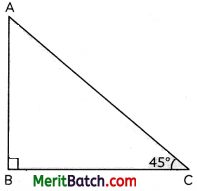AB is the height of the tower which is 125 m. C is the point at which the vehicle standing.
In ∆ABC
tan 45° = $$\frac{AB}{BC}$$
1 = $$\frac{AB}{BC}$$
AB = BC = 125 m
Distance between Tower and vehicle is 125 m.

Question 15.
If cosec A – cot A = 13, then the value of cosec A + cot A is: (1)
(a) $$\frac{1}{12}$$
(b) $$\frac{2}{13}$$
(c) $$\frac{1}{14}$$
(d) $$\frac{1}{13}$$
(d) $$\frac{1}{13}$$

Explanation:
We know that:
cosec2 A – cot2 A = 1
i.e. (cosec A + cot A) (cosec A – cot A) = 1
⇒ cosec A + cot A = $$\frac{1}{cosec A – cot A}$$ = $$\frac{1}{13}$$

Question 16.
If ∆ABC is right-angled at C, then the value of cos (A + B) is: (1)
(a) 0
(b) 2
(c) 1
(d) 4
(a) 0

Explanation:
In ∆ABC,
∠A + ∠B + ∠C = 180°
⇒ ∠A + ∠B + 90° = 180°
⇒ ∠A + ∠B = 90°
Now, cos (A + B) = cos 90° = 0

Question 17.
A wire is in the shape of a circle of radius 21 cm. It is bent to form a square. The length of its side is: (1)
(a) 30 cm
(b) 15 cm
(c) 33 cm
(d) 14 cm
(c) 33 cm

Explanation:
Circumference of circle = Perimeter of the square.
So, 2πr = 4a
⇒ 4a = 2 × $$\frac{22}{7}$$ × 21
⇒ 4a = 132
⇒ a = 33
Hence, the length of each side of square is 33 cm.Question 18.
If the areas of three adjacent faces of a cuboid are X, Y and Z respectively, then the volume of the cuboid is: (1)
(a) XYZ
(b) $$\sqrt{XYZ}$$
(c) $$X \sqrt{YZ}$$
(d) $$Y \sqrt{XZ}$$
(b) $$\sqrt{XYZ}$$

where, l = length, b = breadth and h = height of cuboid.
Then, volume, V = lbh …(i)
Since, XYZ = lb × bh × hl
= l2b2h2
= (lbh)2
or lbh = $$\sqrt{XYZ}$$
Then V = $$\sqrt{XYZ}$$ [using (i)]

DIRECTION: in the question number 19 and 20, a statement of assertion (A) is followed by a statement of reason (R).
Choose the correct option as:
(a) Both assertion (A) and reason (R) are true and reason (R) is the correct explanation of assertion (A)
(b) Both assertion (A) and reason (R) are true and reason (R) is not the correct explanation of assertion (A)
(c) Assertion (A) is true but reason (R) is false.
(d) Assertion (A) is false but reason (R) is true.

Question 19.
Statement A (Assertion): The point (0, 6) lies on y-axis.
Statement R (Reason): The x-coordinate of the point on y-axis is zero. (1)
(a) Both assertion (A) and reason (R) are true and reason (R) is the correct explanation of assertion (A)

Explanation:
We know that the if the point lies on y-axis, its x-coordinate is 0.
The x co-ordinate of the point (0, 6) is zero.
So, Point (0, 6) lies on y-axis.

Question 20.
Statement A (Assertion): Height AB in the figure is 11.56 metres if BC is 20 metres.
Statement R (Reason): tan θ = $$\frac{AB}{BC}$$ = $$\frac{Perpendicular}{base}$$ where θ the angle ∠ACB. (1)
(a) Both assertion (A) and reason (R) are true and reason (R) is the correct explanation of assertion (A)

Explanation:
tan30° = $$\frac{AB}{BC}$$ = $$\frac{AB}{20}$$
AB = $$\frac{1}{\sqrt{3}}$$ × 20 = $$\frac{20}{1073}$$
= 11.56 m

Section – B
(Section B consists of 5 questions of 2 marks each.)

Question 21.
Given that $$\sqrt{5}$$ is an irrational number, prove that 3 + $$\sqrt{5}$$ is irrational.
OR
Show that, points (a, b + c), (b, c + a) and (c, a + b) are collinear. (2)
Suppose 3 + $$\sqrt{5}$$ is a rational number.
Let 3 + $$\sqrt{5}$$ = p
∴ 3 = P – $$\sqrt{5}$$
⇒ 9 = (p – $$\sqrt{5}$$)2 = p2 + 5 – 2$$\sqrt{5}$$p
⇒ 2$$\sqrt{5}$$p = p2 – 4
⇒ $$\sqrt{5}$$ = $$\frac{p^2-4}{2 p}$$
which is a contradiction because Left hand side is an irrational number and right hand side is a rational number.
Hence, 3 + $$\sqrt{5}$$ is an irrational number.
OR
We need to show:
a[(c + a) – (a + b)] + b[(a + b) – (b + c)] + c[(b + c) – (c + a)] = 0
Consider,
a[(c + a) – (a + b)] + b[{a + b) – (b + c)] + c[(b + c) – (c + a)]
= a[c – b] + b[a – c] + c[b – a]
= ac – ab + ba – be + cb – ca
= 0
Thus, the given points are collinear.Question 22.
Two circles with radii of 20 cm and 7 cm, respectively. Find the radius of the circle whose circumference equals the sum of the circumference of the two circles.
OR
The two opposite vertices of a square are (-1, 2) and (3, 2). Find the coordinates of the other two vertices. (2)
Let R be the radius of the circle which has circumference equal to the sum of circumferences of the two circles.
Then according to question,
Radius (r1) of the 1st circle = 20 cm
Radius (r2) of the 2nd circle = 7 cm
Let the radius of the 3rd circle be R.
2πR = 2R(20) + 2π(7)
R = 20 + 7
R = 27 cm
OR
Let ABCD be a square and Let A(- 1, 2) and C(3, 2) be the given two vertices.
Also, let B(x, y) be the unknown vertex.Then, AB = BC
⇒ (x + 1)2 + (y – 2)2 = (3 – x)2 + (2 – y)2
⇒ x2 + 2x + 1 + y2 – 4y + 4 = 9 – 6x + x2 + 4 + y2 – 4y
⇒ 2x + 1 – 4y + 4 = -6x + 9 – 4y + 4
⇒ 8x = 8
⇒ x = 1
Further, in ∆ABC,
AC2 = AB2 + BC2
⇒ (3 + 1)2 + (2 – 2)2 = (x + 1)2 + (y – 2)2 + (3 – x)2 + (2 – y)2
⇒ 16 = 2x2 + 2y2 + 2x + 1 – 4y + 4 – 6x + 9 – 4y + 4
Using x = 1, we get
16 = 2 + 2 y2 + 2 + 1 – 4y + 4 – 6 + 9 – 4y + 4
⇒ 2y2 – 8y = 0
⇒ 2y(y – 4) = 0
⇒ y = 0 or y = 4
Thus, the other two vertices are (1,0) and (1,4).

Question 23.
In the figure, OABC is a rhombus, where O is the origin. The coordinates of A and C are (a, 0) and (s, t) respectively.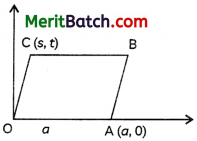(A) Write down the coordinates of B in terms of a, s and t.
(B) Find the length of OC in terms of and t. (2)
(A) Since OC || AB and CB || OA, the coordinates of B are (s + a, t)

(B) Length of OC
= $$\sqrt{(s – 0)^2 + (t – 0)^2}$$
= s2 + t2Question 24.
What is the total surface area of a cuboid with dimensions of 6 cm in length, 2 cm in width, and 2 cm in height? (2)
Given, the dimensions of cuboid are:
Length, l = 6 cm
Width, b = 2 cm
Height, h = 2 cm
Surface area of cuboid = 2(lb + bh + lh)
= 2(6 × 2 + 2 × 2 + 2 × 6)
= 2 (12 + 4 + 12)
= 56 sq.cm.

Question 25.
Find the area of the shaded region in the figure, if ABCD is a square of side 14 cm and APD and BPC are semi-circles. (2)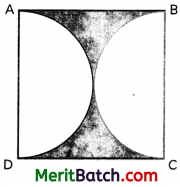Area of the shaded region = Area of square ABCD – 2 × area of semi-circle
= (14 × 14) – 2 × [$$\frac{22}{7}$$ ×(7)2]
= (196 – 2 × 77) cm2
= (196 – 154) cm2
= 42 cm2

Section – C
(Section C consists of 6 questions of 3 marks each.)

Question 26.
Show that 12n cannot end with the digit 0 or 5 for any natural number n.
OR
Which term of the AP: -2, -7, -12, ………., will be -77? Find the sum of this AP up to the term -77. (3)
Given, number is 12n, where n ⊥ N
Now, 12n = (22 × 3)n
Now, for 12n to end with 0, it should have 2 as well as 5 in its prime factors. Also to end with 5, it requires atleast a single multiple of 5 in its prime factors, so 12n cannot end with the digit 0 or 5.
OR
Here, a = – 2 and d = -5
Let nth term of the A.P. be -77. Then,
– 77 = (- 2) + (n – 1) (- 5)
⇒ 5(n – 1) = 75
⇒ n – 1 = 15, or n = 16
⇒ Sum up to the term (-77)
= $$\frac{n}{2}$$ [a + 1]
= $$\frac{16}{2}$$ [(-2) + (-77)]
= 8 × (- 79)
= -632

Question 27.
Determine the number of real roots of the equation: (x2 + 1)2 – x2 = 0 (3)
(x2 + 1)2 – x2 = 0
x4 + 2x2 + 1 – x2 = 0
x4 + x2 + 1 = 0
Put t = x2. Then
t2 + t + 1 = 0
Comparing this equation with ax2 + bx + c = 0, we get,
a = 1, b = 1, c = 1
So, D = b2 – 4ac = 1 – 4 = -3 < 0
which shows that given equation has no real roots.

Question 28.
5 books and 7 pens together cost ₹ 434, whereas 7 books and 5 pens together cost ₹ 550, find the total cost of 1 book and 2 pens. (3)
Let, the cost of 1 book be ₹ x and cost of 1 pen be ₹ y.
5x + 7y = 434 …(i)
7x + 5y = 550 …(ii)
Add equations (i) and (ii) we get
12x + 12y = 984
x + y = 82 …(iii)
Subtract equation (ii) from (i), we get
2x- 2 y = 116
x – y = 58 …(iv)
Now, add equations (iii) and (iv), we get
2x = 140 x = 70
y = 70 – 58 = 12
Hence, the cost of 1 book is ₹ 70 and the cost of 2 pens is ₹ 24.

Question 29.
Prove that:
$$\frac{\tan A}{1+\sec A}-\frac{\tan A}{1-\sec A}=2 cosec A$$
OR
IF sin = $$\frac{12}{13}$$, find the value of
$$\frac{\sin ^2 \theta-\cos ^2 \theta}{2 \sin \theta \cos \theta}-\frac{1}{\tan ^2 \theta}$$. (3)OR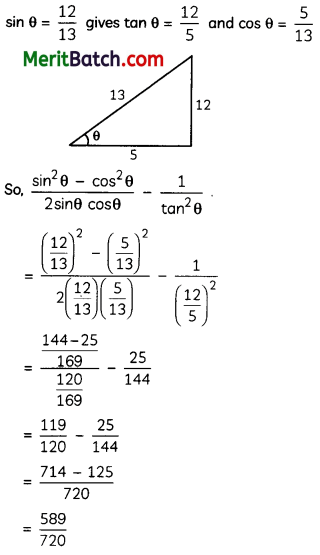Question 30.
A carpenter cuts a wooden cone into three parts A, B and C by two planes parallel to the base as shown in the figure.
The heights of the three parts are equal.
(A) Find the ratio of the volumes of parts A, B and C
(B) Find the ratio of the base areas of parts A, B and C
(C) If the volume of the original cone is 540 cu cm, find the volume of part B. (3)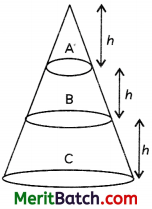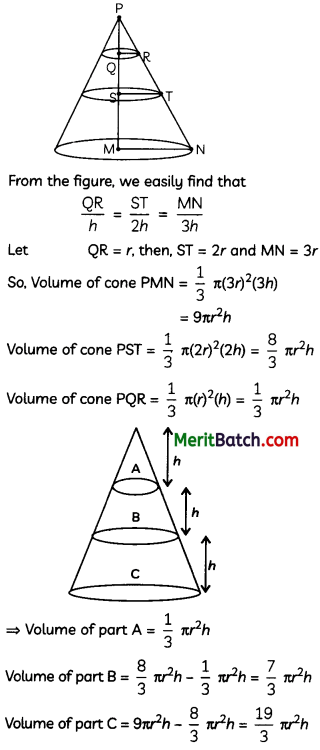(A) Ratio of volumes of parts A, B and C
= $$\frac{1}{3}=\frac{7}{3}=\frac{19}{3}$$ i.e. 1:7:19

(B) Ratio of Base area of part A, B and C are
πr2 : π(2r)2 : π(3r)2 i.e. 1 : 4 : 9

(C) Volume of cone PMN = 9πr2h = 540
⇒ πr2h = 60
So, volume of part B = $$\frac{7}{3}$$ πrh = $$\frac{7}{3}$$ × 60 cm3
= 140 cm3

Question 31.
Find the mean and median of the following data: (3)

 Age (in years) less than 10 less than 20 less than 30 less than 40 less than 50 Frequency 5 15 20 24 30

Put x2 = y to make partial fractions

 Mass Frequency (fi) xi fixi c.f. 0-10 5 5 25 5 10-20 10 15 150 15 20-30 5 25 125 20 30-40 4 35 140 24 40-50 6 45 270 30 Σfi = 30 710

Mean = $$\frac{\Sigma f_i x_i}{\Sigma f_i}=\frac{710}{30}$$
= 23.67 (approx)

For median $$\frac{N}{2}$$ = $$\frac{30}{2}$$ = 15.

Cumulative frequency just greater than 15 is 20, which belongs to class 20-30.
Median class is 20-30
Now, Median = $$l+\frac{\left(\frac{\mathrm{N}}{2}-c f\right)}{f} \times h$$
= 20 + $$\frac{15-15}{5}$$ × 10
= 20 + 0
= 20

Section – D
(Section D consists of 4 questions of 5 marks each.)

Question 32.
If the zeroes of polynomial p(x) = ax3 + 3bx2 + 3cx + d are in A.P., then prove that 2b3 – 3abc + a2d = 0.
OR
From the top of a tower ‘h’ metres high, angle of depression of two objects which are in line with foot of the tower are a and fa (fa > a). Find the distance between the two objects. (5)
Let, the zeros of the given polynomial be p, q, r. As the roots are in A.P., then it can be assumed as p – k, p, p + k, where k is the common difference.OR
Let AB represent the tower of height ‘hi metres.
Also, let P and Q be the positions of the two objects on the ground such that their distances from the tower AB is b and a, respectively.Let ∠BPA = α and ∠BQA = β
We need to determine the distance PQ.
From ∆QAB, we have
tan β = $$\frac{h}{QA}$$ ⇒ QA = h cot β
From ∆PAB, we have
tan α = $$\frac{h}{PA}$$ ⇒ PA = h cot α
Further
PQ = PA – QA
= h cot α – h cot β, or h(cot α – cot β)
Thus, the distance between two objects is, h(cot α – cot β).Question 33.
Two tangents TP and TQ are drawn to a circle with centre O from an external point T. Prove that:
(A) TP = TQ
(B) ∠PTQ = 2∠OPQ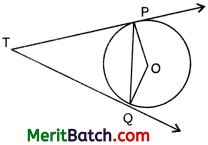OR
Prove that the area of the semi-circle drawn on the hypotenuse of a right-angled triangle is equal to the sum of the areas of the semi-circles drawn on the other two- sides of the triangle. (5)
(A) Join OT.
Consider As OPT and OQTHere
∠P = ∠Q = 90° (each)
OT = OT (common)
So, by RHS congruence criterion,
∆OPT = ∆OQT
⇒ TP = TQ

(B) In ∆OPQ,
∠OQP = ∠OPQ (∵ OP = OQ)
= $$\frac{1}{2}$$ [180° -∠POQ]
= $$\frac{1}{2}$$ [∠PTQ]
[∵ In quad. OPTQ, ∠P = ∠Q = 90°
So, ∠POQ + ∠PTQ = 180°]
⇒ ∠PTQ = 2∠OPQ
OR
Given: ∆ABC, right angled at B.
Here, O,
O’ and O” are the mid-points of AC, AB and BC, respectively
Area of semicircle at AC
= $$\frac{1}{2} \times \pi(O A)^2$$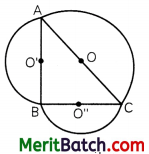= $$\frac{1}{2} \pi\left(\frac{\mathrm{AC}}{2}\right)^2$$
Area of semicircle at ABQuestion 34.
The following quadratic polynomials have zeroes; find them and confirm the relationship between the zeroes and the coefficients.
(A) x2 – 2x – 8
(B) 4s2 – 4s + 1
(C) 6x2 – 3 – 7x
(D) 4u2 + 8u
(E) t2 – 15 (5)
x2 – 2x – 8
Comparing given polynomial with general form
ax2 + bx + c,
We get a = 1, b = – 2 and c = -8
We have, x2 – 2x – 8
= x2 – 4x + 2x – 8
= x(x – 4) + 2(x – 4)
= (x-4)(x+2)
Equating this equal to 0 will find value of 2 zeroes of this polynomial,
(x – 4)(x + 2) = 0
⇒ x = 4, – 2 are two zeroes.
Sum of zeroes = 4 – 2 = 2
$$\frac{-\text { Coefficient of } x}{\text { Coefficient of } x^2}=\frac{-b}{a}=\frac{-(-2)}{1}$$
Product of zeroes = 4 × – 2 = – 8
$$\frac{-\text { Coefficient of } x}{\text { Coefficient of } x^2}=\frac{c}{a}=\frac{-8}{1}$$

(B) 4s2 – 4s + 1
We have, 4s2 – 4s + 1
= 4s2 – 2s – 2s + 1
= 2s(2s – 1) – 1(2s – 1)
= (2s – 1)(2s – 1)
Equating this equal to 0 will values of 2 zeroes of this polynomial.
⇒ (2s – 1)(2s – 1) = 0
⇒ s = $$\frac{1}{2}$$, $$\frac{1}{2}$$
Therefore, two zeroes of this polynomial are $$\frac{1}{2}$$,$$\frac{1}{2}$$
Sum of zeroes = $$\frac{1}{2}$$ + $$\frac{1}{2}$$ = 1
= $$\frac{-(-1)}{1} \times \frac{4}{4}=\frac{-(-4)}{4}$$
= $$=\frac{-b}{a}=\frac{-\text { Coefficient of } x}{\text { Coefficient of } x^2}$$

Product of Zeroes = $$\frac{1}{2} \times \frac{1}{2}-\frac{1}{4}=\frac{c}{a}$$
= $$\frac{\text { Constant term }}{\text { Coefficient of } x^2}$$

(C) 6x2 – 3 – 7x
We have, 6x2 – 3 – 7x
= 6x2 – 7x – 3
= 6x2 – 9x + 2x – 3
= 3x(2x – 3) + 1(2x – 3)
= (2x – 3)(3x + 1)
Equating this equal to 0 will find values of 2 zeroes of this polynomial.
⇒ (2x – 3)(3x + 1) = 0
⇒ x = $$\frac{3}{2}$$, $$\frac{-1}{3}$$
Therefore, two zeroes of this polynomial are $$\frac{3}{2}$$, $$\frac{-1}{3}$$
Sum of zeroes = $$\frac{3}{2}$$ + $$\frac{-1}{3}$$ = $$\frac{9-2}{6}$$ = $$\frac{7}{6}$$
= $$\frac{-(-7)}{6}$$ = $$\frac{-b}{a}$$= $$\frac{-\text { Coefficient of } x}{\text { Coefficient of } x^2}$$

Product of Zeroes = $$\frac{3}{2} \times \frac{-1}{3}=\frac{-1}{2}=\frac{c}{a}$$
= $$\frac{\text { Constant term }}{\text { Coefficient of } x^2}$$(D) 4u2 + 8u
Here, a = 4, fa = 8 and c = 0
4u2 + 8 u = 4 u(u + 2)
Equating this equal to 0 will find values of 2 zeroes of this polynomial.
⇒ 4 u(u + 2) = 0
⇒ u = 0,-2
Therefore, two zeroes of this polynomial are 0,-2
Sum of zeroes = 0 – 2 = – 2
= $$\frac{-2}{1} \times \frac{4}{4}=\frac{-8}{4}=\frac{-b}{a}$$
= $$\frac{-\text { Coefficient of } x}{\text { Coefficient of } x^2}$$

Product of Zeroes = 0 × – 2 = 0
= $$\frac{0}{4}=\frac{c}{a}$$
= $$\frac{\text { Constant term }}{\text { Coefficient of } x^2}$$

(E) t2 – 15
We have, t2 – 15
⇒ t2 = 15
⇒ t= ±$$\sqrt{15}$$
Therefore, two zeroes of this polynomial are $$\sqrt{15}$$,$$\sqrt{15}$$
Sum of zeroes = $$\sqrt{3}$$ + (-$$\sqrt{3}$$) = 0
= $$\frac{0}{1}=\frac{-b}{a}$$ = $$\frac{-\text { Coefficient of } x}{\text { Coefficient of } x^2}$$

Product of Zeroes = $$\sqrt{15}$$ + $$\sqrt{-15}$$ = -15
= $$\frac{-15}{1}=\frac{c}{a}$$ = $$\frac{\text { Constant term }}{\text { Coefficient of } x^2}$$

Question 35.
The midpoints of the sides AB, BC, CD, and DA in the quadrilateral ABCD are P,Q,R, and S, respectively. Show that PQRS is a parallelogram.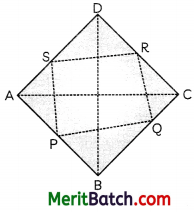To Prove: PQRS is a parallelogram
Proof: In ∆DAC,
= $$\frac{DS}{SA}=\frac{DR}{RC}=1$$
[∵ S and R are mid-points of AD and DC]
⇒ SR || AC ,…(i) [by converse of B.P.T.]
In ∆BAC,
= $$\frac{DS}{SA}=\frac{DR}{RC}=1$$
[∵ P and Q are mid-points of AB and BC]
⇒ PQ || AC ….(ii) [by converse of B.P.T.]
From (i) and (ii), we get
⇒ SR || PQ ….(iii)
Similarly, join B to D and PS || QRSection – E (12 marks)
(Case Study Based Questions)
(Section E consists of 3 questions. Alt are compulsory.)

Question 36.
A crane stands on a level ground. It is represented by a tower ABCD, of height 11 m and a jib BR. The jib is of length 20 m and can rotate in a vertical plane about B. A vertical cable, RS, carries a load S. The diagram shows current position of the jib, cable and load.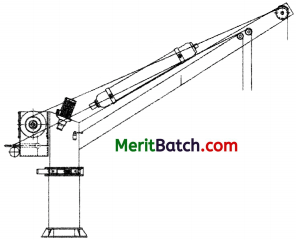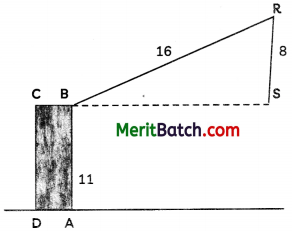On the basis of the above information, answer the following questions:
(A) Find the length BS. (1)
(B) Find the angle that the jib, BR, makes with the horizontal. (1)
(C) In the diagram below, the jib BR, has been rotated and the length RS is increased. The load S is now on the ground at a point 8 m from A. Find the measure of the angle BRS and also find the angle through which the jib has been rotated.OR
Find the length by which RS has increased. (2)
(A) In ∆BSR, using Pythagoras theorem, we have
BR2 = BS2 + SR2
(16)2 = BS2 + 82
BS2 = 256 – 64
= 192
BS = 13.9 m

(B) In ∆BRS
sin B = $$\frac{SR}{BR}$$ = $$\frac{8}{16}$$
∠B = 30°

C) In ∆BRS,
∠BRS = 180° – 90° – 30°
= 180° – 120°
= 60°

In ∆BRT,
cos ∠BRT = $$\frac{BR}{BT}$$
= $$\frac{8}{16}$$ = $$\frac{1}{2}$$
∠BRT = 60°
Angle of rotation = 60° – 30° = 30°

OR

In ∆BRT, using Pythagoras theorem, we have
BR2 = BT2 + RT2
⇒ (16)2 = BT2 + 82
⇒ BT2 = 256 – 64 = 192
⇒ BT = 13.9
Hence, increase in length
= 13.9 – 8 = 5.9 cm
From all the given options, the value nearest to 5.9 m is 6 m.

Question 37.
NITI aayog has tasked their statistical officer to create a model for farmers to be able to predict their produce output based on various factors.
To test the model out, the officer picked a local farmer who sells apples to check various factors like weight, bad apples, half-cooked, green vs red etc.
A box containing 250 apples was opened and each apple was weighed.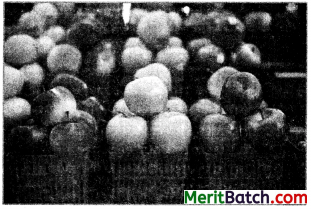The distribution of the masses of the apples is given in the following table:

 Marks Frequency 80-100 20 100-120 60 120-140 70 140-160 p 160-180 60

On the basis of the above information, answer the following questions:
(A) Find the value of p. (1)
(B) Find the mean mass of the apples. (1)
(C) Find the upper limit of the median class.
OR
Find the modal mass of the apples. (2)
(A) Since, total apples are 250.
Then
20 + 60 + 70 + p + 60 = 250
p = 250 – 210 =40

(B)

 Marks fi xi fixi 80-100 20 90 1800 100-120 60 110 6600 120-140 70 130 9100 140-160 40 150 6000 160-180 60 170 10200 250 33700

Then, mean = $$\frac{33700}{250}$$ = 134.8 g

(C)

 Marks Frequency c.f. 80-100 20 20 100-120 60 80 120-140 70 150 140-160 40 190 160-180 60 250 N = 250

Then, $$\frac{N}{2}$$ = $$\frac{250}{2}$$ = 125
So, cumulative frequency just greater than 125 is 150, which belong to class 120-140. So. median class is 120-140.
Hence, upper limit of median class is 140.OR

Here, modal class is 120 – 140
Then, l = 120, h = 20
f0 = 60, f1 = 70, f2 = 40
Then, M0 = $$l+\left(\frac{f_1-f_0}{2 f_1-f_0-f_2}\right) \times h$$
= $$120+ \frac{70-60}{140-60-40} \times 20$$
= 120 + $$\frac{200}{40}$$
= 125 g

Question 38.
A ticket machine in a car park takes ₹ 1 coin and ₹ 2 coin. A ticket cost f7 3. The probability that the machine will accept a particular ₹ 1 coin is 0.9 and that it will accept a particular ₹ 2 coin is 0.8On the basis of the above information, answer the following questions:
(A) Find the probability that jayant will not get a ticket. (1)
(B) Pritam has one ₹ 1 coins and two coins. Find the probability that Pritam will get a ticket. (1)
(C) Urmila put one ₹ 1 coin and one ₹ 2 coin into the machine. Find the probability that the machine will not accept either of these coins.
OR
Jayant only has three ₹ 1 coins. Find the probability that the machine accept all these coins. Also find the probability that the machine will not accept a particular ₹ 1 coin. (2)